## Multiplying Polynomials

Multiplying polynomials is a bit more challenging than adding and subtracting polynomials. We must use the distributive property to multiply each term in the first polynomial by each term in the second polynomial. We then combine like terms. We can also use a shortcut called the FOIL method when multiplying binomials. Certain special products follow patterns that we can memorize and use instead of multiplying the polynomials by hand each time. We will look at a variety of ways to multiply polynomials.

## Multiplying Polynomials Using the Distributive Property

To multiply a number by a polynomial, we use the distributive property. The number must be distributed to each term of the polynomial. We can distribute the $2$ in $2\left(x+7\right)$ to obtain the equivalent expression $2x+14$. When multiplying polynomials, the distributive property allows us to multiply each term of the first polynomial by each term of the second. We then add the products together and combine like terms to simplify.

### How To: Given the multiplication of two polynomials, use the distributive property to simplify the expression.

1. Multiply each term of the first polynomial by each term of the second.
2. Combine like terms.
3. Simplify.

### Example 4: Multiplying Polynomials Using the Distributive Property

Find the product.

$\left(2x+1\right)\left(3{x}^{2}-x+4\right)$

### Solution

$\begin{array}{cc}2x\left(3{x}^{2}-x+4\right)+1\left(3{x}^{2}-x+4\right) \hfill & \text{Use the distributive property}.\hfill \\ \left(6{x}^{3}-2{x}^{2}+8x\right)+\left(3{x}^{2}-x+4\right)\hfill & \text{Multiply}.\hfill \\ 6{x}^{3}+\left(-2{x}^{2}+3{x}^{2}\right)+\left(8x-x\right)+4\hfill & \text{Combine like terms}.\hfill \\ 6{x}^{3}+{x}^{2}+7x+4 \hfill & \text{Simplify}.\hfill \end{array}$

### Analysis of the Solution

We can use a table to keep track of our work, as shown in the table below. Write one polynomial across the top and the other down the side. For each box in the table, multiply the term for that row by the term for that column. Then add all of the terms together, combine like terms, and simplify.

 $3{x}^{2}$ $-x$ $+4$ $2x$ $6{x}^{3}\\$ $-2{x}^{2}$ $8x$ $+1$ $3{x}^{2}$ $-x$ $4$

### Try It 4

Find the product.

$\left(3x+2\right)\left({x}^{3}-4{x}^{2}+7\right)$

Solution

## Using FOIL to Multiply Binomials

A shortcut called FOIL is sometimes used to find the product of two binomials. It is called FOIL because we multiply the first terms, the outer terms, the inner terms, and then the last terms of each binomial.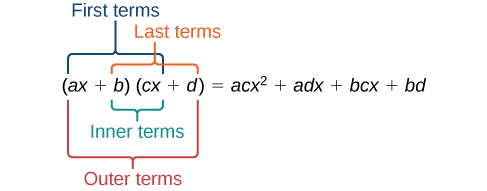The FOIL method arises out of the distributive property. We are simply multiplying each term of the first binomial by each term of the second binomial, and then combining like terms.

### How To: Given two binomials, use FOIL to simplify the expression.

1. Multiply the first terms of each binomial.
2. Multiply the outer terms of the binomials.
3. Multiply the inner terms of the binomials.
4. Multiply the last terms of each binomial.
6. Combine like terms and simplify.

### Example 5: Using FOIL to Multiply Binomials

Use FOIL to find the product.

### Solution

Find the product of the first terms.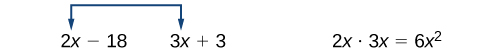Find the product of the outer terms.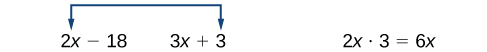Find the product of the inner terms.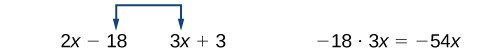Find the product of the last terms.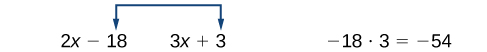$\begin{array}{cc}6{x}^{2}+6x - 54x - 54\hfill & \text{Add the products}.\hfill \\ 6{x}^{2}+\left(6x - 54x\right)-54\hfill & \text{Combine like terms}.\hfill \\ 6{x}^{2}-48x - 54\hfill & \text{Simplify}.\hfill \end{array}$

### Try It 5

Use FOIL to find the product.

$\left(x+7\right)\left(3x - 5\right)$

Solution